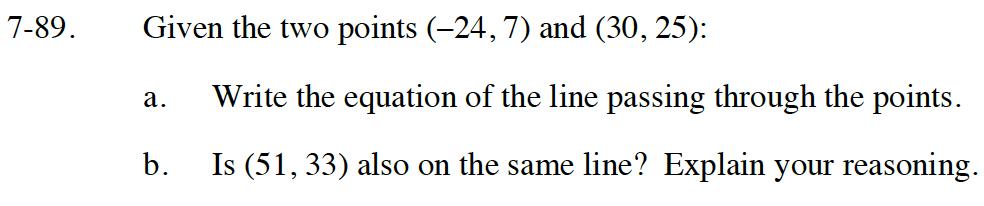### Home > CC4 > Chapter 7 > Lesson 7.2.1 > Problem7-89

7-89.
1. Given the two points (–24, 7) and (30, 25): 7-89 HW eTool (Desmos) Homework Help ✎

1. Write the equation of the line passing through the points.

2. Is (51, 33) also on the same line? Explain your reasoning.Use the slope-intercept form (y = mx + b). Substitute the slope (m), and one point (−24, 7) or (30, 25) for x and y to solve for b.

$y=\frac{1}{3}x+15$

Use the eTool below for part (b).
Click the link at right for the full version of the eTool: INT1 7-89 HW eTool